# Report Logic Examples

The following examples illustrate how reports are constructed.

Note: AND and OR operators always have at least two child nodes. The nodes are either fields or Drag a field here placeholders.

(1 or ((2 or 3) and (4 or 5)) or (6 and 7)) and 8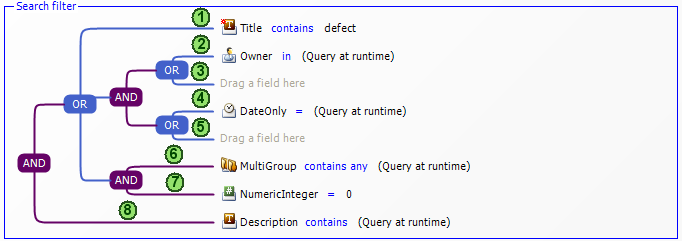1 and 2 and 3 and 4 and 5 and (6 or 7)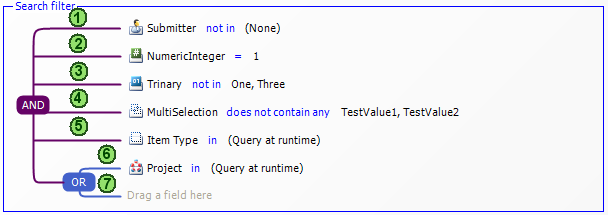(1 and 2) or 3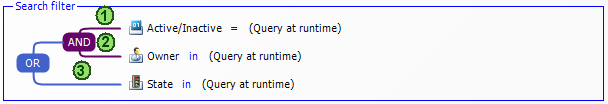1 and (2 or 3)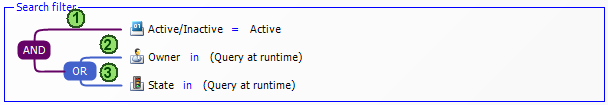(1 or 2 or 3) and 4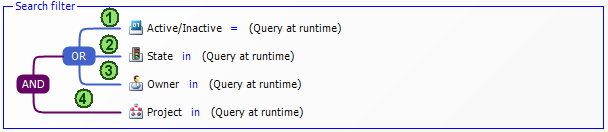(1 and (2 or 3)) or 4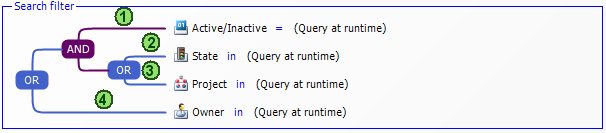Report Operators

Basic Conditions